## HIbernate BigDecimal映射到MySql float字段出现问题

RunACoding 发布于 2016/10/13 17:09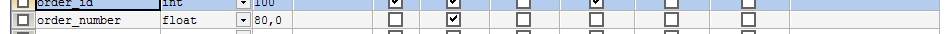hibernate中配置

<property name="orderNumber" type="java.math.BigDecimal">
<column name="order_number" scale="0" length="80" precision="80" sql-type="NUMERIC(80,0)"/>
</property>

0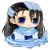0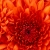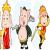0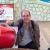The maximum number of digits for DECIMAL is 65, but the actual range for a given DECIMAL column can be constrained by the precision or scale for a given column. When such a column is assigned a value with more digits following the decimal point than are permitted by the specified scale, the value is converted to that scale. (The precise behavior is operating system-specific, but generally the effect is truncation to the permissible number of digits.)0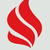mysql的float类型保存的不是精确值。

http://dev.mysql.com/doc/refman/5.6/en/floating-point-types.html